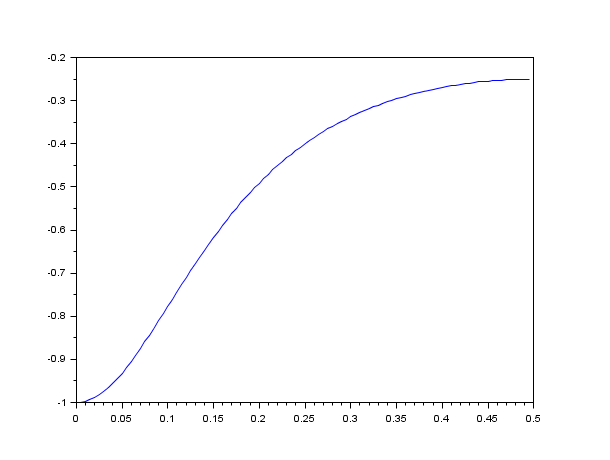Change language to:
English - Français - 日本語 - Português -

See the recommended documentation of this function

Справка Scilab >> Signal Processing > Filters > group

# group

group delay for digital filter

### Syntax

```[tg [,fr]] = group(npts, H) (call with a siso transfert function)
[tg [,fr]] = group(npts, C) (call with a vector of transfert functions, cascaded second order representation)

[tg [,fr]] = group(npts, F) (call with the vector of an FIR filter coefficients)
[tg [,fr]] = group(npts, a1i, a2i, b1i, b2i) (call with 4 vectors of numbers, cascaded second order Deczky representation)```

### Arguments

npts

integer : number of points desired in calculation of group delay

a1i

in coefficient, polynomial, rational polynomial, or cascade polynomial form this variable is the transfer function of the filter. In coefficient polynomial form this is a vector of coefficients (see below).

a2i

in coeff poly form this is a vector of coeffs

b1i

in coeff poly form this is a vector of coeffs

b2i

in coeff poly form this is a vector of coeffs

tg

values of group delay evaluated on the grid fr

fr

grid of normalized frequency values where group delay is evaluated

### Description

Calculate the group delay of a digital filter with transfer function h(z).

The filter specification can be in coefficient form, polynomial form, rational polynomial form, cascade polynomial form, or in coefficient polynomial form.

In the coefficient polynomial form the transfer function is formulated by the following expression

`h(z)=prod(a1i+a2i*z+z**2)/prod(b1i+b2i*z+z^2)`

### Examples

```s = poly(0, "s");
h_cont = syslin("c", 1/(s-10));
h = ss2tf(cls2dls(tf2ss(h_cont), 0.1));
[tg, fr] = group(100, h);
plot(fr, tg)```Report an issue << fsfirlin Filters hilbert >>